# AP Chemistry : Principles of Oxidation-Reduction Reactions

## Example Questions

← Previous 1

### Example Question #1 : Principles Of Oxidation Reduction Reactions

Methane combusts in the presence of oxygen according to the following reaction: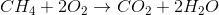Which of the following statements is true concerning the reaction?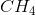will be the limiting reagent

Oxygen has a charge of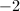throughout the entire reaction

Carbon is oxidized in the reaction

Oxygen is oxidized in the reaction

Carbon has an initial oxidation state of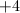Carbon is oxidized in the reaction

Explanation:

By comparing the oxidation number of an atom as a reactant and its oxidation number as a product, we can determine if the atom has been oxidized or reduced. In increase in oxidation number indicates a loss of electrons, or oxidation. A decrease in oxidation number signals a gain of electrons, or reduction.

For electrochemistry, you should familiarize yourself with the traditional oxidation states of hydrogen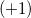, halogens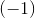, oxygen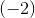, and elemental atoms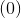Carbon is initially in the form of methane, meaning that it is attached to four hydrogen atoms. The molecule is neutral, and each hydrogen has an oxidation number of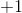. Carbon must have an initial oxidation state ofin order to balance the molecular charge.

In the a product, carbon is attached to two oxygens, each with a charge of. Again, the molecule is neutral, so carbon must balance these charges. This means that carbon's final oxidation state is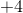.

Since carbon went from an oxidation state ofto, we can conclude that carbon has been oxidized in the reaction.

### Example Question #2 : Principles Of Oxidation Reduction Reactions

Which of the following reactions involves the transfer of electrons?

Redox

Decomposition

Combination

Neutralization

Redox

Explanation:

Redox reactions occur when one species is oxidized—loses electrons—and another is reduced—gains electrons. Thus, this is the only one of the given options that involves the transfer of electrons.

### Example Question #3 : Principles Of Oxidation Reduction Reactions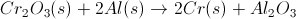Chromium is the oxidizing reagent for this reaction.

Aluminum is oxidized during the reaction.

Chromium is oxidized during the reaction

This reaction is an example of a single displacement reaction.

Chromium is oxidized during the reaction

Explanation:

In Cr2O3, Cr has a charge of +3. The chromium metal product is neutral, and therefore has a charge of 0. So, Cr is reduced from +3 to 0.

Remember, OIL RIG. Oxidation Is Loss (of electrons) and Reduction Is Gain (of electrons).

### Example Question #4 : Principles Of Oxidation Reduction Reactions

Consider the following reaction: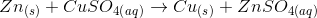Which of the following statements is true?

Zinc is reduced and copper is oxidized

Sulfur is reduced and copper is reduced

Zinc is oxidized and copper is reduced

Sulfur is oxidized and copper is reduced

Zinc is oxidized and copper is reduced

Explanation:

For any oxidation-reduction reaction, the oxidized atom will lose electrons and the reduced atom will gain electrons. Zinc is originally in elemental form, so it has an initial oxidation state of zero. As a product, it has a charge of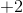. This means that zinc has been oxidized in the reaction; its oxidation number increased due to a loss of electrons.

Copper originally has an oxidation state of, but changes to its elemental form as a product. This gives copper a final oxidation state of zero. Since it has gained electrons, copper has been reduced.

Sulfur remains in the sulfate complex ion, which retains its overallcharge throughout the reaction. It is neither oxidized, nor reduced.

### Example Question #1 : Principles Of Oxidation Reduction Reactions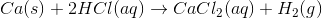In this reaction, electrons are transferred from ___________ to ___________.

calcium . . . hydrogen

hydrogen . . . calcium

calcium . . . chlorine

chlorine . . . hydrogen

hydrogen . . . chlorine

calcium . . . hydrogen

Explanation:

A transfer of electrons indicates an oxidation-reduction reaction. The element losing electrons is oxidized, while the element gaining electrons is reduced.

Calcium is initially a neutral solid, but as a product has a charge of. Calcium's charge increased, meaning it must have lost electrons.

Hydrogen initially has a charge of, but as a product is a neutral diatomic gas. Hydrogen's charge decreased, meaning it must have gained electrons.

We can thus conclude that calcium was oxidized and hydrogen was reduced, indicating that electrons were transferred from calcium to hydrogen.

The charge on chlorine remainsthroughout the entire reaction.

### Example Question #1 : Principles Of Oxidation Reduction Reactions

In the following reaction, which compound is being oxidized?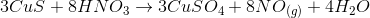Sulfur

Nitrate

Hydrogen

Copper

No oxidation takes place

Sulfur

Explanation:

Hydrogen doesn't change. Cu2+ doesn't change (partnered with S2- then with SO42-). Sulfur goes from S2- and S6+(paired with 6 O2- with a 2– charge), showing an oxidation. Nitrogen goes from N5+ to N2+ meaning it was reduced.

### Example Question #7 : Principles Of Oxidation Reduction Reactions

Consider the following reaction: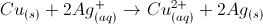What is the oxidizing agent, and what is the reducing agent?

Silver is the oxidizing agent and copper is the reducing agent

No redox chemistry occurs

Silver is the reducing agent and there is no oxidizing agent

Silver is the reducing agent and copper is the oxidizing agent

Silver is the oxidizing agent and there is no reducing agent

Silver is the oxidizing agent and copper is the reducing agent

Explanation:

Let's break down the reaction into two separate reactions: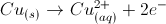and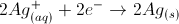We can see that copper loses electrons, while silver gains electrons. Recall that oxidation is loss and reduction is gain, with regard to electrons. Copper is oxidized and silver is reduced.

However, this question asks for the oxidizing agent and reducing agent. Recall that the oxidizing agent is reduced, while the reducing agent is oxidized. Since copper is oxidized, it is the reducing agent. Similarly, since silver is reduced, it is the oxidizing agent.

### Example Question #1 : Principles Of Oxidation Reduction Reactions

What does oxidation mean?

Gain of protons

Loss of neutrons

Loss of electrons

Gain of electrons

Loss of protons

Loss of electrons

Explanation:

Think "OIL RIG" Oxidation Is Loss of electrons, Reduction Is Gain of electrons. The loss and gain of electrons is what changes the charge of the atom or molecule. In redox reactions, electrons are transferred. Changing the number of protons changes the identity of the element ,which is not the case. Since chemical reactions involve valence electrons, the nucleus is unchanged.

### Example Question #9 : Principles Of Oxidation Reduction Reactions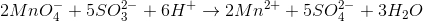Which statement about the reaction equation is correct?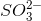is the reducing agentis reduced

This is a combustion reaction

This is a hydrolysis reaction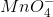is the reducing agentis the reducing agent

Explanation:is converted to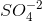. Thus, an additional oxygen has been added to the original molecule, thuswas oxidized. The substance that is oxidized is also called the reducing agent because it causes the reduction of the other molecule to which the oxygen originally was bound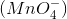, which is the oxidizing agent.

### Example Question #10 : Principles Of Oxidation Reduction Reactions

Oomycetes of the genus Phytophthora are capable of causing economic damage by destroying crops. The name Phytopthora from the Greek phyton = plant, and phthora = destruction literally means plant-destroyer. However, chemists may battle their destructive effects.

The compound named copper(II) carbonate can be used to destroy the plant-destroyer, and can be produced as shown in the following equation: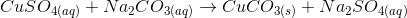What is the net ionic equation for the given reaction that produces copper(II) carbonate?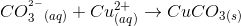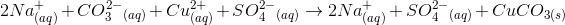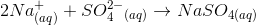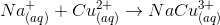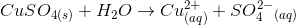Explanation:

### An ionic equation is a chemical equation in which ionic compounds in an aqueous solution are written as dissociated ions.

A complete ionic equation shows all the ions present in the solution (which includes both the reactive and spectator ions), as the dissociated ions. The complete ionic equation for this problem's reaction is:It is worth noting that in a complete ionic equation, it becomes readily apparent which ions are involved in the oxidation-reduction reaction, and which ions are the spectator ions (those that do not change during the reaction). For example since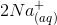and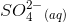are present on both sides of the reaction, they are spectator ions that go through the reaction unchanged. Also because the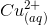and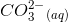are only present as dissolved ions on the reactants side of the equation and form the solid precipitate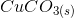on the product side, they are the reactive species in this chemical reaction.

The net ionic equation shows only the reactive ions in solution and the product(s) formed by them. Therefore a net ionic equation can most simply be made by looking at the complete ionic equation and omitting the spectator ions as shown below:

Net ionic equation:← Previous 1